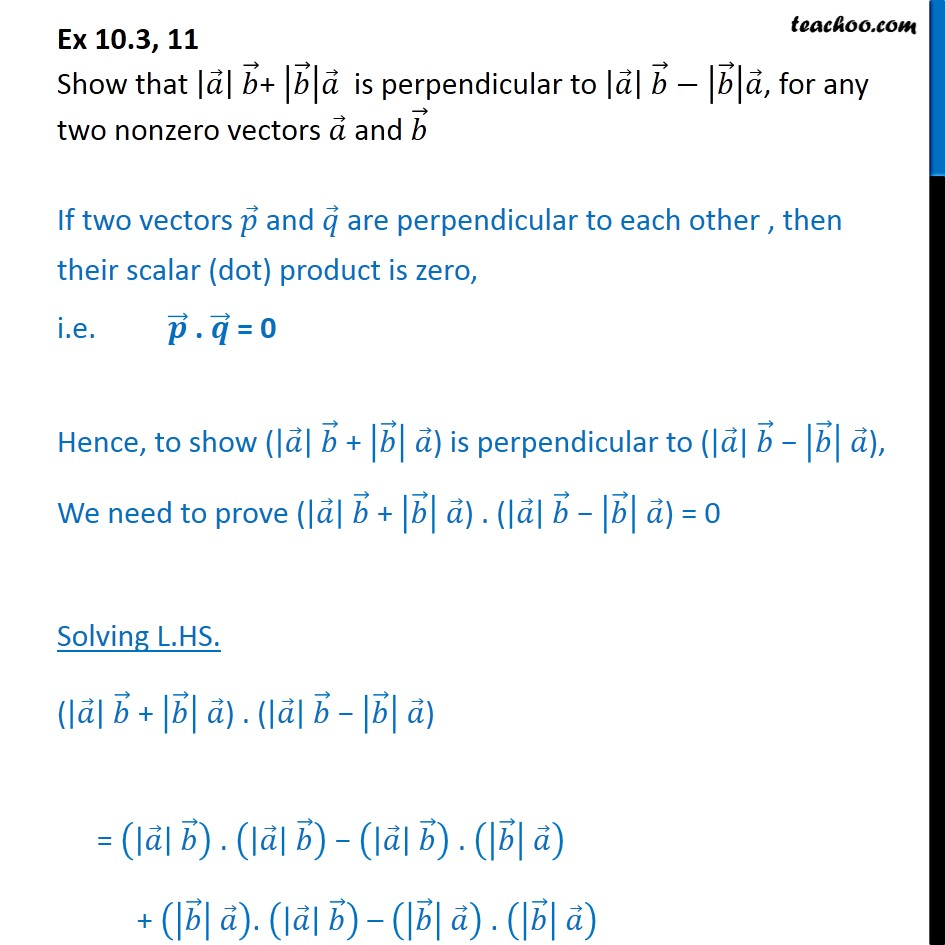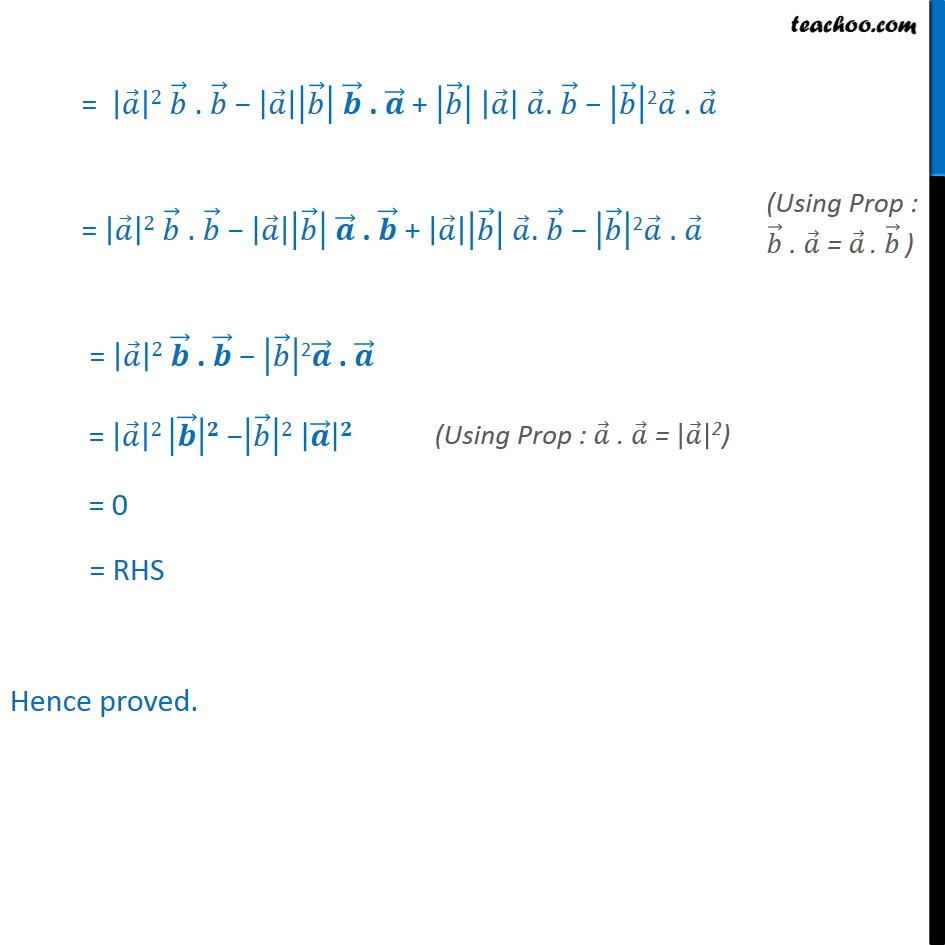Subscribe to our Youtube Channel - https://you.tube/teachoo

1. Chapter 10 Class 12 Vector Algebra
2. Serial order wise
3. Ex 10.3

Transcript

Ex 10.3, 11 Show that + is perpendicular to , for any two nonzero vectors and If two vectors and are perpendicular to each other , then their scalar (dot) product is zero, i.e. . = 0 Hence, to show ( + ) is perpendicular to ( ), We need to prove ( + ) . ( ) = 0 Solving L.HS. ( + ) . ( ) = . . + . . = 2 . . + . 2 . = 2 . . + . 2 . = 2 . 2 . = 2 2 = 0 = RHS Hence proved.

Ex 10.3